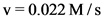# Calculate the initial rate for the formation of C \rm C at 25?

Calculate the initial rate for the formation of C \rm C at 25 ?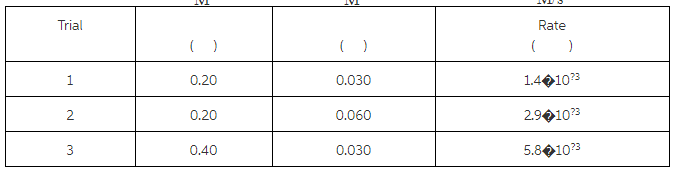A =2, B =1 k[A]^2

strong textConcepts and reason
Rate:
The rate of a chemical reaction is defined as the change in concentration of substance (reactant or product) per unit time. It is expressed in M/S.

Order:
Order of the reaction is a number, which indicates the raise of concentration of the substance.
Significant figures:
The term significant figures refer to the meaningful digits in it.

Fundamentals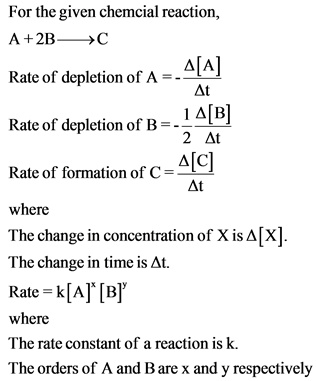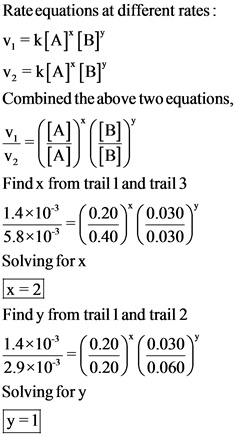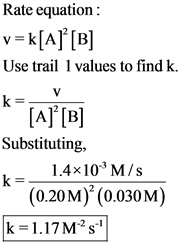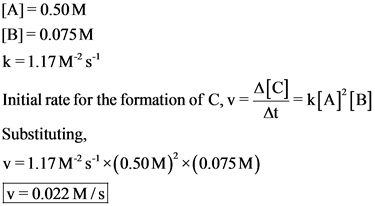The initial rate for the formation of reactant, C at 25 °C is